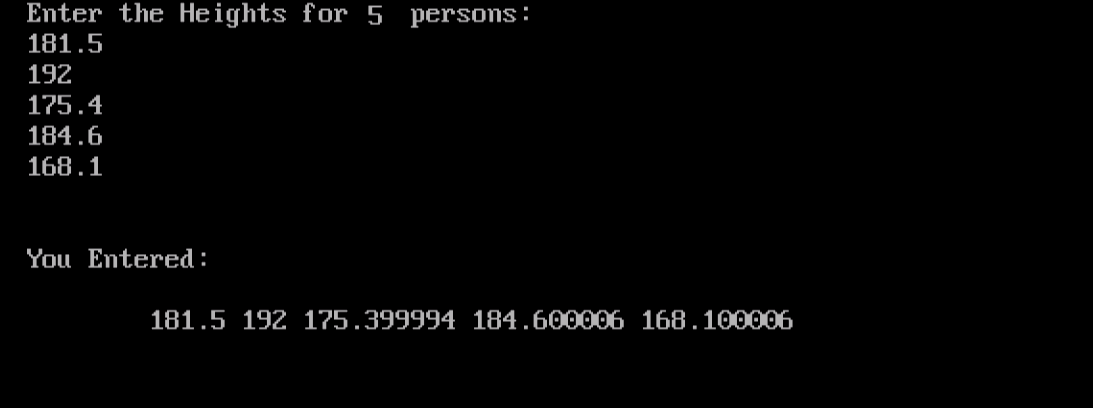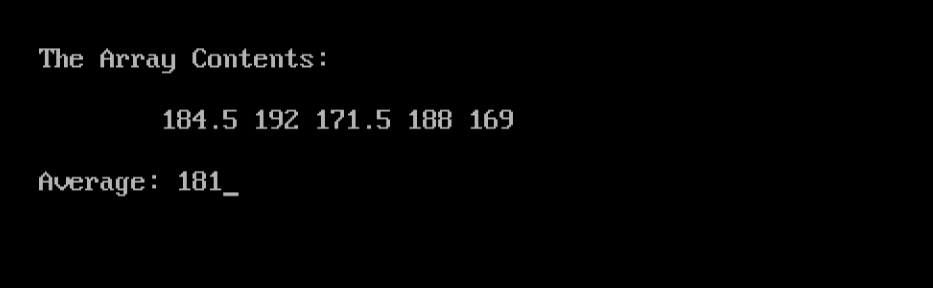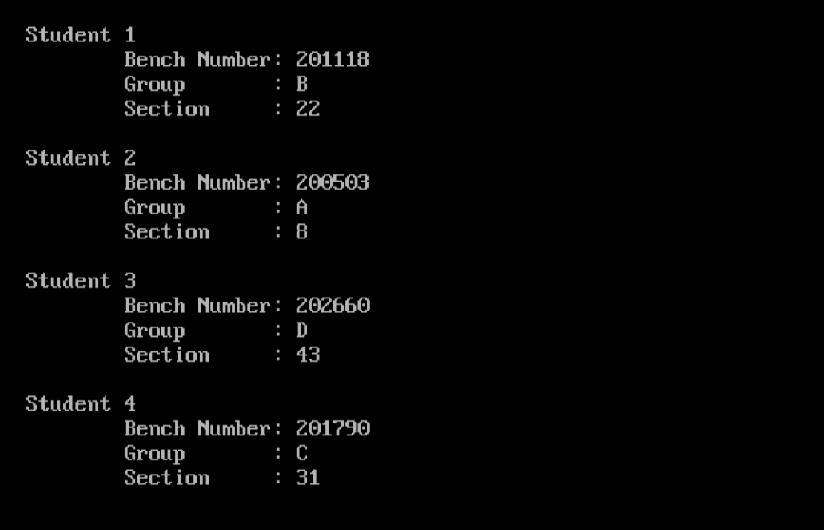# Learn about Arrays in C++ Programming

0
2802When talking about Structures, I mentioned that Structures are used to group items of different data types together into a larger entity that has a name. Arrays have a similar concept; they are also used to group data items, but those items must be of the same data type. Defining and Using Arrays will be the subject of this article; so, let’s start.

What are Arrays?
An Array is a collection of objects that belong to the same data type. While individual data items in a structure are called member variables (and accessed using the dot operator), the ones in an array are called array elements (and are accessed via their position in the array, i.e. index).

Declaring Arrays
To use an array, it must be declared (defined) first. Defining an array is similar to declaring a variable, except one extra thing: the size (number of elements) of the array must also be mentioned.

Syntax

`DATATYPE ARRAYNAME[SIZE];`

Where:
DATATYPE is the type of the data that will be stored in the array elements. It could be a basic data type like int, float, char, etc, or a complex one like structures and classes (Classes will be explained in details later in this course when talking about Object Oriented Programming).
ARRAYNAME is the name of array. It could be any valid C++ identifier.
SIZE is a positive integer that represents the number of elements in the array.

Accessing Array Elements
While a member variable in a structure is accessed using the dot operator, an element of an array is accessed by its position (index). For an array with n elements, the first element will be at index 0, the second element will be at index 1, and the last element will be at index n-1.

Example
The following program prompts the user to enter the heights for 5 persons, and stores them in an array. The program then prints the entered data.

```#include<iostream.h>
#include<conio.h>
void main()
{
clrscr();
float heights;
cout << "Enter the Heights for 10 persons:\n";
for (int i=0;i<5;i++)
cin >> heights[i];
cout << "\n\nYou Entered:\n\n\t";
for (int j=0;j<5;j++)
cout << heights[j] << " ";
getch();
}
```

When executed, the program should behave like the following:Initializing Arrays
If the array elements are known in advance, an entire array could be initialized as follows:

`float heights [] = { 181.5, 192, 175.4, 184.6, 168.1 };`

Notice that we didn’t specify the array size; this is simply because the size of the array could be easily identified from the number of initializing values (which is 5 in this case).Arrays as Arguments to Functions
Arrays could be passed as arguments to functions. In this case, the memory address of the array is passed to the function, so that the function actually works with the original array, not a copy from it. Does this sound familiar?  This is similar to passing arguments by reference (refer to the Functions article).

Example
Re-write the previous program to calculate the average of the entered heights.
Consider the following modified version:

```#include<iostream.h>
#include<conio.h>
float average(float h)
{
float total = 0;
for (int i=0;i<5;i++)
total += h[i];
}
void main()
{
clrscr();
float heights [] = { 184.5, 192, 171.5, 188, 169 };
cout << "\n\nThe Array Contents:\n\n\t";
for (int j=0;j<5;j++)
cout << heights[j] << " ";
cout << "\n\nAverage: " << average (heights);
getch();
}
```

This program should print the following output:In this program, both heights (in the main() function), and h (in the average() function) refer to the same array.

Arrays of Custom Types
As I told you above, the data type of an array could be either basic or complex data type. In this section, we are going to see how to use Arrays of structures.

Example
Back to the University Students example that we discussed when talking about Structures. This time, we are going to define an array of that structure type.

```#include <iostream.h>
#include <conio.h>
struct student
{
long BN;
char group;
char section;
};
void main()
{
clrscr();
student ARRAY[] = { { 201118, 'B', 22 }, { 200503, 'A', 8 },
{ 202660, 'D', 43 }, { 201790, 'C', 31 } };
for (int i=0;i<4;i++)
cout << "\nStudent " << i+1 << endl
<< "\tBench Number: "  << ARRAY[i].BN << endl
<< "\tGroup       : "  << ARRAY[i].group << endl
<< "\tSection     : "  << int(ARRAY[i].section) << endl;
getch();
}
```

When executed, this program should give the following output:Summary